Ex 12.1

Chapter 12 Class 6 Ratio and Proportion
Serial order wise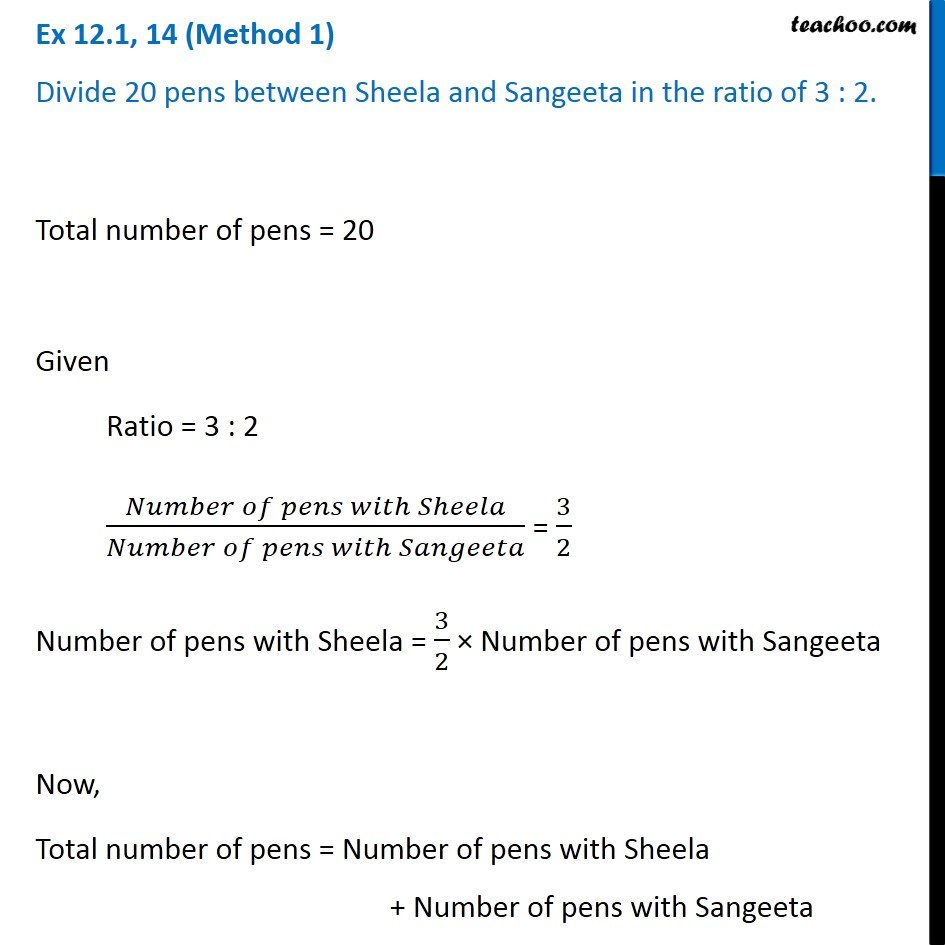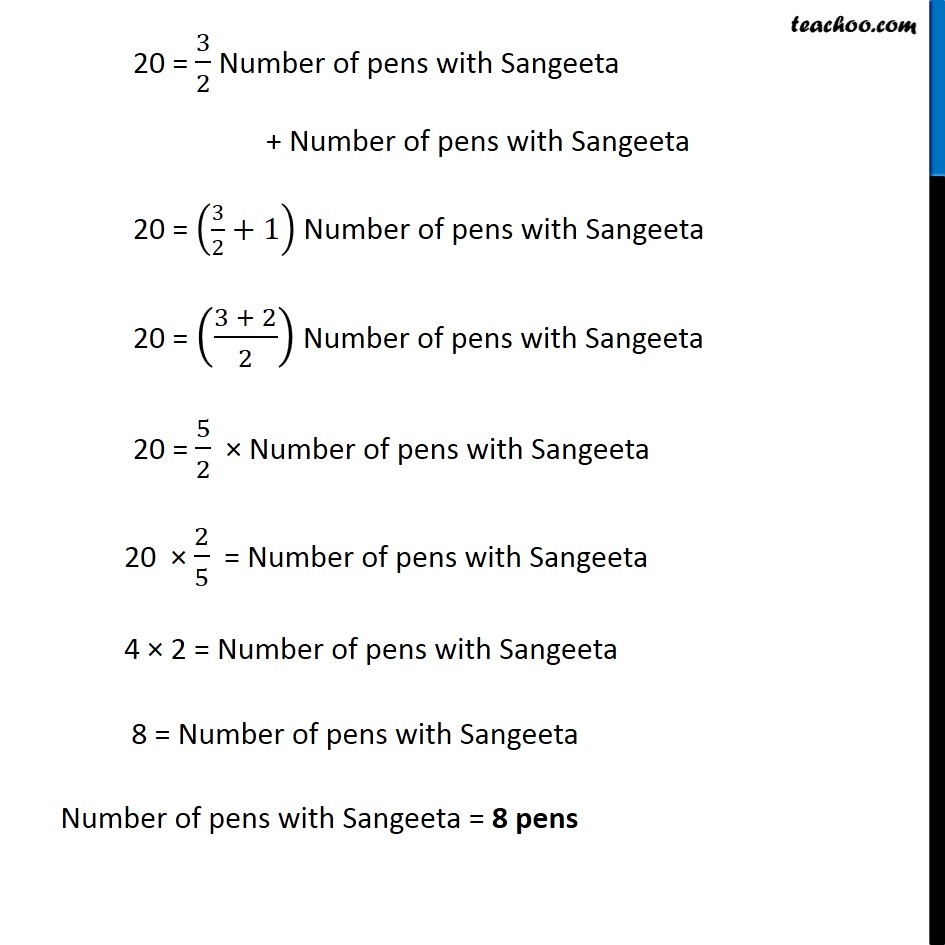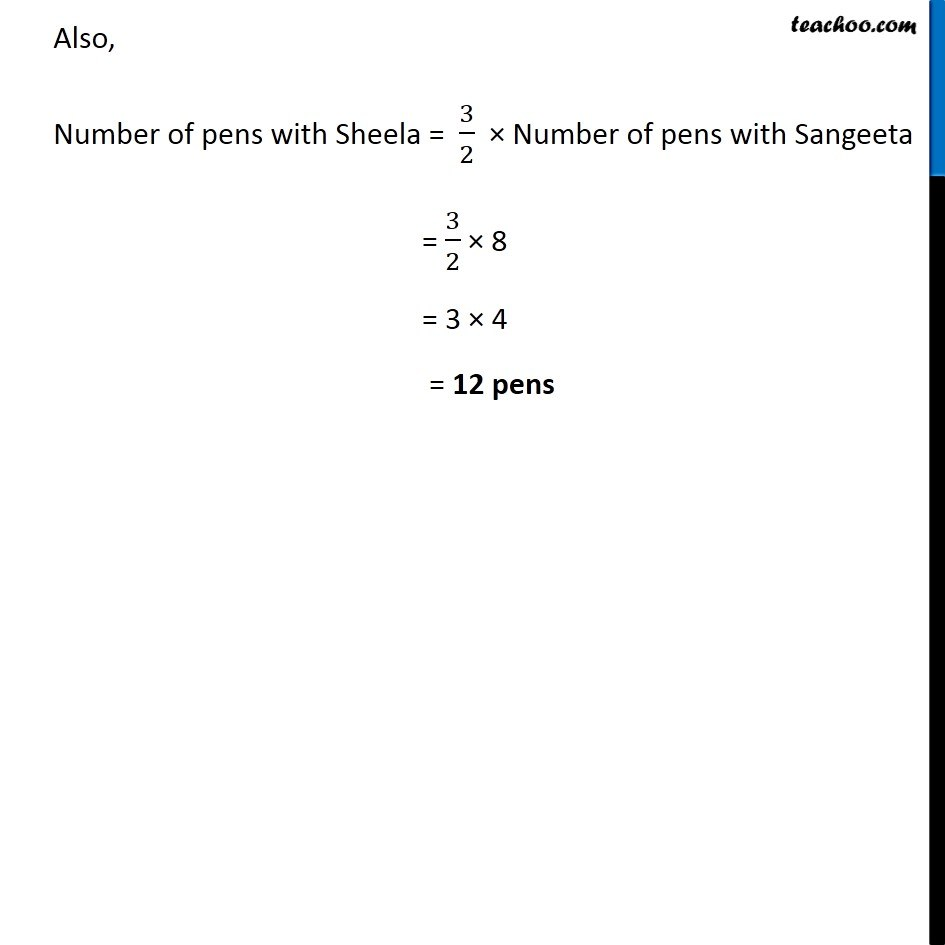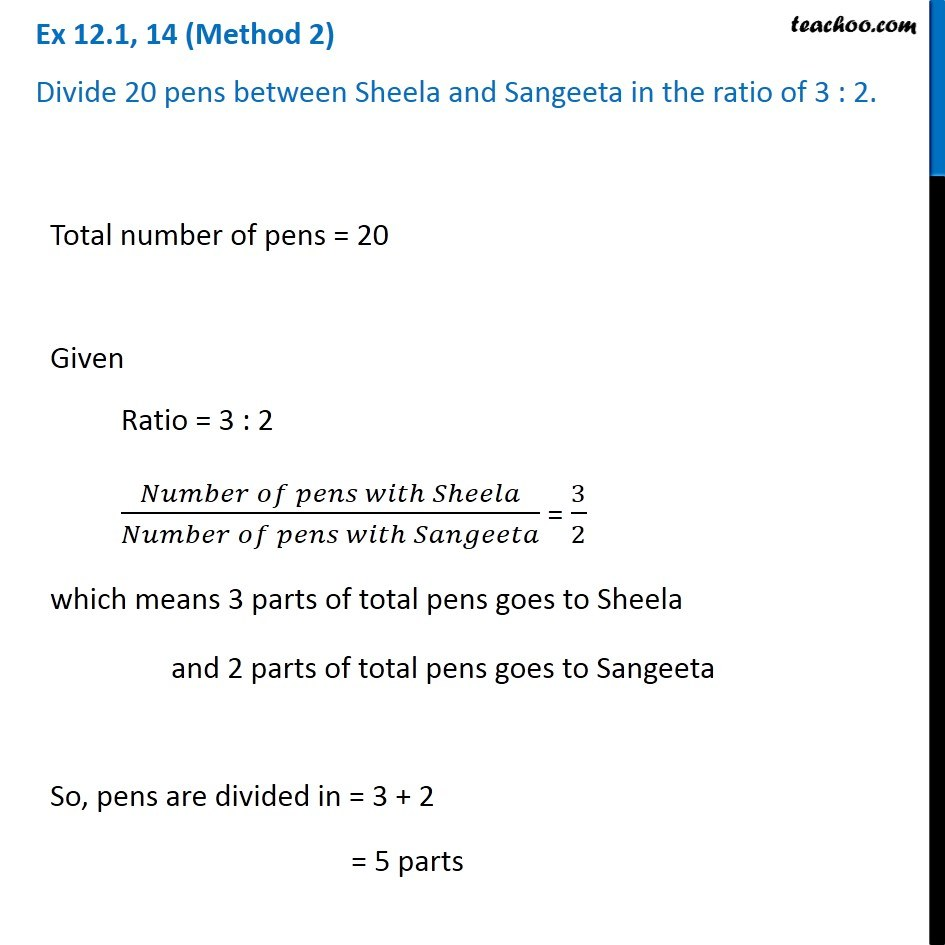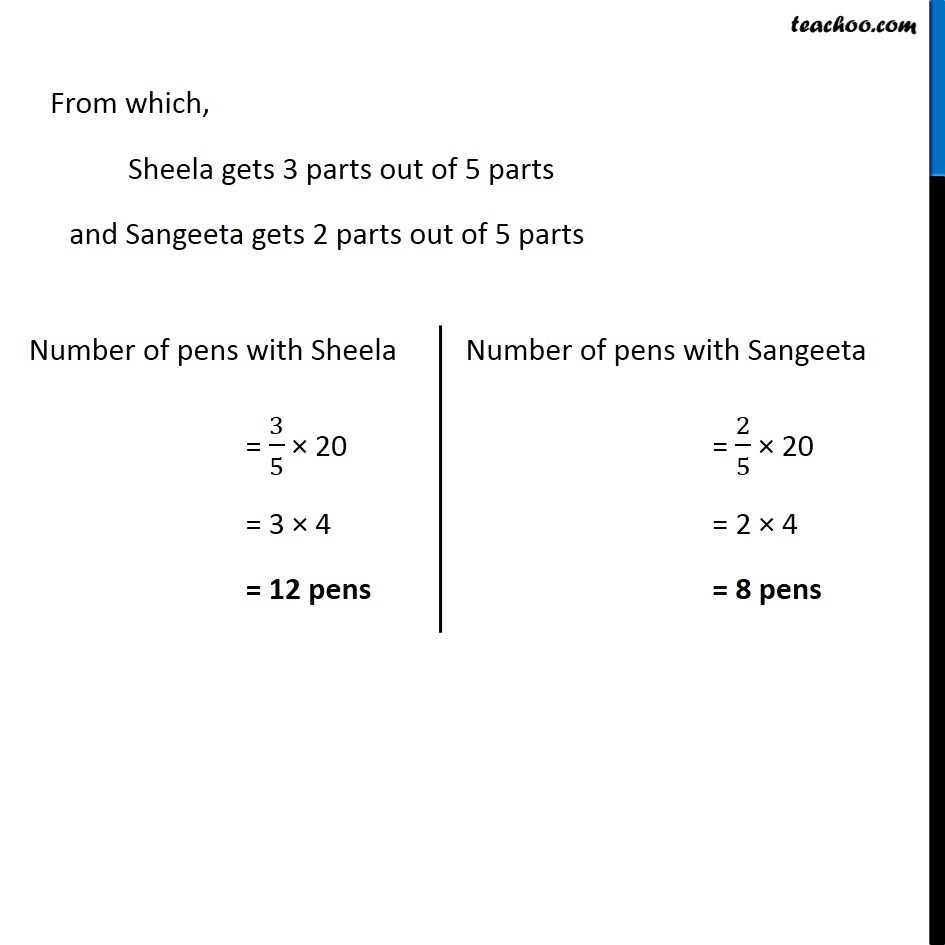Learn in your speed, with individual attention - Teachoo Maths 1-on-1 Class

### Transcript

Ex 12.1, 14 (Method 1) Divide 20 pens between Sheela and Sangeeta in the ratio of 3 : 2. Total number of pens = 20 Given Ratio = 3 : 2 (𝑁𝑢𝑚𝑏𝑒𝑟 𝑜𝑓 𝑝𝑒𝑛𝑠 𝑤𝑖𝑡ℎ 𝑆ℎ𝑒𝑒𝑙𝑎)/(𝑁𝑢𝑚𝑏𝑒𝑟 𝑜𝑓 𝑝𝑒𝑛𝑠 𝑤𝑖𝑡ℎ 𝑆𝑎𝑛𝑔𝑒𝑒𝑡𝑎) = 3/2 Number of pens with Sheela = 3/2 × Number of pens with Sangeeta Now, Total number of pens = Number of pens with Sheela + Number of pens with Sangeeta 20 = 3/2 Number of pens with Sangeeta + Number of pens with Sangeeta 20 = (3/2+1) Number of pens with Sangeeta 20 = ((3 + 2)/2) Number of pens with Sangeeta 20 = 5/2 × Number of pens with Sangeeta 20 × 2/5 = Number of pens with Sangeeta 4 × 2 = Number of pens with Sangeeta 8 = Number of pens with Sangeeta Number of pens with Sangeeta = 8 pens Also, Number of pens with Sheela = 3/2 × Number of pens with Sangeeta = 3/2 × 8 = 3 × 4 = 12 pens Ex 12.1, 14 (Method 2) Divide 20 pens between Sheela and Sangeeta in the ratio of 3 : 2.Total number of pens = 20 Given Ratio = 3 : 2 (𝑁𝑢𝑚𝑏𝑒𝑟 𝑜𝑓 𝑝𝑒𝑛𝑠 𝑤𝑖𝑡ℎ 𝑆ℎ𝑒𝑒𝑙𝑎)/(𝑁𝑢𝑚𝑏𝑒𝑟 𝑜𝑓 𝑝𝑒𝑛𝑠 𝑤𝑖𝑡ℎ 𝑆𝑎𝑛𝑔𝑒𝑒𝑡𝑎) = 3/2 which means 3 parts of total pens goes to Sheela and 2 parts of total pens goes to Sangeeta So, pens are divided in = 3 + 2 = 5 parts From which, Sheela gets 3 parts out of 5 parts and Sangeeta gets 2 parts out of 5 parts Number of pens with Sheela = 3/5 × 20 = 3 × 4 = 12 pens Number of pens with Sangeeta = 2/5 × 20 = 2 × 4 = 8 pens From which, Sheela gets 3 parts out of 5 parts and Sangeeta gets 2 parts out of 5 parts Number of pens with Sheela = 3/5 × 20 = 3 × 4 = 12 pens Number of pens with Sangeeta = 2/5 × 20 = 2 × 4 = 8 pens